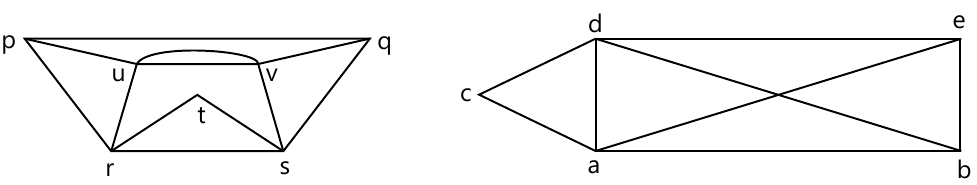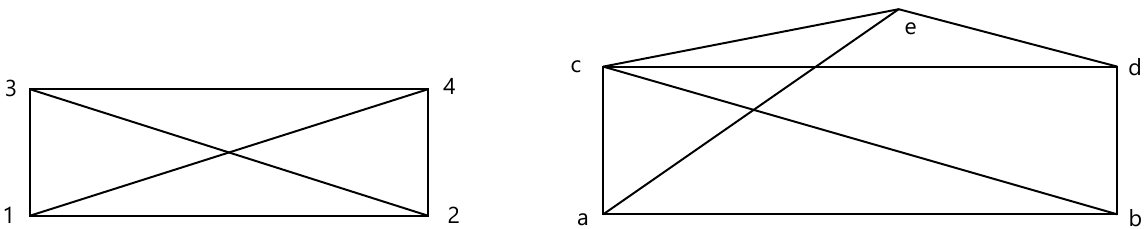MORE IN Discrete Structures
MU Computer Engineering (Semester 3)
Discrete Structures
May 2014
Total marks: --
Total time: --
INSTRUCTIONS
(1) Assume appropriate data and state your reasons
(2) Marks are given to the right of every question
(3) Draw neat diagrams wherever necessary

1 (a) Prove that 8n - 3n is a multiple of 5 by mathematical induction, n ≥ 1
5 M
1 (b) Show that if a relation on set A is transitive an irreflexive, then it is asymmetric.
5 M
1 (c) Function f(x)=(4x+3)/(5x-2). Find f-1
5 M
1 (d) What is the total number of vertices in a full binary tree with 20 leaves?
5 M

2 (a) Let f(x)=x+2, g(x)=x-2 and h(x)=3x for all x ? R. (R is the set of real number). Find (i) f° g° h (ii)h° g° f (iii) f° f° f
8 M
2 (b) Let R be a relation on the set of integers Z defined by a Rb if and only if a=m(mod 5). Prove that R is an equivalence relation. Find Z/R.
8 M
2 (c) Show that A x (B ∩ C) = (A x B) ∩ (A x B)
4 M

3 (a) Let A={1,2,3,4) and R={ (1,2), (2,3), (3,4), (2,1) }. Find the transitive closure using Warshall's algorithm.
6 M
3 (b) Consider the lattices L1={1,2,4}, L2={1,3,9} under divisibility. Draw the lattice L1 x L2.
7 M
3 (c) Solve the recurrence relation an = -3(an-1 + an-2) - an-3 with a0=5, a1= -9 and a2=15
7 M

4 (a) show that a group G is abelian if and only if (ab)2=a2b2 for all a,b ∈ G
6 M
4 (b) Prove that the set G={1,2,3,4,5,6} is an abellian group under multiplication modulo 7.
6 M
4 (c) Find the generating function for the following series
(i) {0,1,2,3,4,??}
(ii) {1,2,3,4,5 . . . . . .}
(iii) {2,2,2,2,2 . . . . . . .}
(iv) {0,0,0,1,1,1,1, . . . . . . . .}
8 M

5 (a) Let $H=\left[\begin{array}{cccccc}1 & 0 & 0 \\1 & 1 & 0 \\0 & 1 & 1 \\1 & 0 & 0 \\0 & 1 & 0 \\0 & 0 & 1\end{array}\right]$ be parity check matrix.
Decode the following words relative to maximum likelyhood decoding function.
(i) 011001 (ii) 101011 (iii) 111010 (iv) 110110
8 M
5 (b) Determine the Eulerian and Hamiltonian path, if exist, in the following graphs :-6 M
5 (c) Let G be the set of real numbers and let Let G be the set of real numbers and let a*b=ab/2. Showthat (G,*) is a abellian group
6 M

6 (a)8 M
6 (b) Use the laws of logic to determine the following expression as tautology or contradiction.
[p^ (p ⇒ q)] ⇒ q
6 M
6 (c) Draw the Hasse Diagram of the following:
(a) D105 (b) D72
6 M

More question papers from Discrete Structures